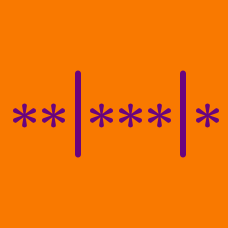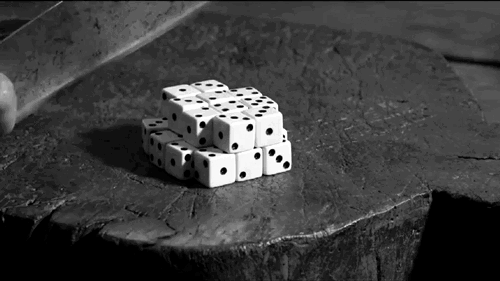Probability

# Distribution into Bins: Level 4 Challenges

$\large \sum_{k=2}^{2016}(-1)^k{2016 \choose k}k^{2015} = \ ?$What is the probability that a roll of 5 distinct dice yields a sum of 17)? Round to 4 significant figures.

How many necklaces can be formed by joining together 12 green beads and 3 red beads?

Note: All beads have to be used. Rotations and reflections are considered identical.

How many 6-digit numbers can be formed using exactly 4 different digits?

Seven identical eggs are being put into a $15 \times 1$ egg box. However no more than two eggs can be put in a row. For example, one possible arrangement is E-E----EE-E--EE.

How many possible arrangements are there for the eggs?

Note: The egg box always stays in the same orientation so reflections count.

How many subsets of $\{1,2,3, \ldots, 14\}$ contain no pair of consecutive integers?

Details and assumptions

The empty set is a subset of every set.

×

Problem Loading...

Note Loading...

Set Loading...